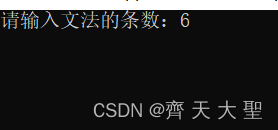+关注继续查看

# 一、值传递和指针传递的概念

## （一）值传递

1、形参只能是变量，实参可以是常量、变量或表达式。在被定义的函数中，必须指定形参的类型。

2、实参与形参的个数应一样，类型应一致。字符型和整型可以互相通用。

4、实参传递给形参是实参的值，形参变量在未出现函数调用时，并不占用内存，只在调用时才占用。调用结束后，将释放内存。值传递过程中参数的数据传递是单向的，数据(实参的值)从实参传递形参，而不能由形参传回实参。执行一个被调用函数时，形参的值如果发生改变，并不会改变主调函数中的实参的值。

4、形参如同公式中的符号，实参就是符号具体的值，在调用过程前必须得到赋值；调用过程就是实现形参与实参的结合，把实参的值通过调用传递给形参，相当于把值代入公式进行计算。值传递的本质就是表达式。

## （二）地址传递

1、实参必须是是变量，也就是保证可以重新被赋值或者初始化。在被定义的函数中，必须指定形参的类型。

2、实参与形参的个数应一样，类型应一致。字符型和整型可以互相通用。

3、实参传递给形参的是实参变量的地址，函数调用过程中，并不为形参开辟存储空间，也就是说地址传递过程中形参和实参共用实参的存储空间，对形参的操作就是对实参本身的操作。

## （三）值传递，地址传递的区别

1、值传递实参向形参传递的是实参的值，而地址传递传递的却是实参的地址。

2、值传递在函数调用过程中会为形参重新开辟空间，形参与实参分别占用不同的地址空间，而地址传递，形参和实参共用同一内存地址。

# 二、C语言函数参数的传递规律

## （一）普通类型变量的参数传递

1. void fun_of_value(int par_value)
2. {
3. printf("In function, the Address of the VALUE = %p\n", &par_value);
4. printf("In function, the Value of the VALUE = %d\n\n\n", par_value);
5. }
6. int main(void)
7. {
8. int arg_value = 10;
9. printf("In main, the Address of the VALUE = %p\n", &arg_value);
10. printf("In main, the Value of the VALUE = %d\n", arg_value);
11. fun_of_value(value);
12. printf("After function, the Address of the VALUE = %p\n", &arg_value);
13. printf("After function, the Value of the VALUE = %d\n", arg_value);
14. }
void fun_of_value(int par_value)
{
printf("In function, the Address of the VALUE = %p\n", &par_value);
printf("In function, the Value of the VALUE = %d\n\n\n", par_value);
}
int main(void)
{
int arg_value = 10;
printf("In main, the Address of the VALUE = %p\n", &arg_value);
printf("In main, the Value of the VALUE = %d\n", arg_value);
fun_of_value(value);
printf("After function, the Address of the VALUE = %p\n", &arg_value);
printf("After function, the Value of the VALUE = %d\n", arg_value);
}

1. In main, the Address of the VALUE = 0028feec
2. In main, the Value of the VALUE = 10
3. In function, the Address of the VALUE = 0028fed0
4. In function, the Value of the VALUE = 10
In main, the Address of the VALUE = 0028feec
In main, the Value of the VALUE = 10
In function, the Address of the VALUE = 0028fed0
In function, the Value of the VALUE = 10## （二）指针变量的参数传递

### 1、普通指针的作为参数

1. void fun_of_point(int *par_point)
2. {
3. printf("In function, the Address of the POINT = %p\n", &par_point);
4. printf("In function, the Value of the POINT = %p\n\n\n", par_point);
5. }
6. int main(void)
7. {
8. int  value = 10;
9. int *arg_point = &value;
10. printf("The Addreess of the value = %p\n", &value);
11. printf("In main, the Address of the POINT = %p\n", &arg_point);
12. printf("In main, the Value of the POINT = %p\n", arg_point);     // 打印参数的值
13. fun_of_point(point);
14. return EXIT_SUCCESS;
15. }
void fun_of_point(int *par_point)
{
printf("In function, the Address of the POINT = %p\n", &par_point);
printf("In function, the Value of the POINT = %p\n\n\n", par_point);
}
int main(void)
{
int  value = 10;
int *arg_point = &value;
printf("The Addreess of the value = %p\n", &value);
printf("In main, the Address of the POINT = %p\n", &arg_point);
printf("In main, the Value of the POINT = %p\n", arg_point);	 // 打印参数的值
fun_of_point(point);
return EXIT_SUCCESS;
}

1. The Addreess og the value 0028feec
2. In main, the Address of the POINT = 0028fee8
3. In main, the Value of the POINT = 0028feec
4. In function, the Address of the POINT = 0028fed0
5. In function, the Value of the POINT = 0028feec
The Addreess og the value 0028feec
In main, the Address of the POINT = 0028fee8
In main, the Value of the POINT = 0028feec
In function, the Address of the POINT = 0028fed0
In function, the Value of the POINT = 0028feec### 2、函数指针的参数

1. void function(void){}
2. void fun_of_fun_point(void(*par_pfun)(void))
3. {
4. printf("In function, the Address of the FUNCTION = %p\n", &par_pfun);
5. printf("In function, the Value of the FUNCTION = %p\n\n\n", par_pfun);
6. }
7. int main(void)
8. {
9. void (*arg_pfun)(void);
10. arg_pfun = /*&*/function;
11. printf("The Address of the FUNCTION = %p\n", function);
12. printf("In main, the Address of the FUNCTION = %p\n", &arg_pfun);
13. printf("In main, the Value of the FUNCTION = %p\n", arg_pfun);
14. fun_of_fun_point(arg_pfun);
15. return EXIT_SUCCESS;
16. }
void function(void){}
void fun_of_fun_point(void(*par_pfun)(void))
{
printf("In function, the Address of the FUNCTION = %p\n", &par_pfun);
printf("In function, the Value of the FUNCTION = %p\n\n\n", par_pfun);
}
int main(void)
{
void (*arg_pfun)(void);
arg_pfun = /*&*/function;
printf("The Address of the FUNCTION = %p\n", function);
printf("In main, the Address of the FUNCTION = %p\n", &arg_pfun);
printf("In main, the Value of the FUNCTION = %p\n", arg_pfun);
fun_of_fun_point(arg_pfun);
return EXIT_SUCCESS;
}

1. The Address of the FUNCTION = 004016c9
2. In main, the Address of the FUNCTION = 0028feec
3. In main, the Value of the FUNCTION = 004016c9
4. In function, the Address of the FUNCTION = 0028fed0
5. In function, the Value of the FUNCTION = 004016c9
The Address of the FUNCTION = 004016c9
In main, the Address of the FUNCTION = 0028feec
In main, the Value of the FUNCTION = 004016c9
In function, the Address of the FUNCTION = 0028fed0
In function, the Value of the FUNCTION = 004016c9与前面采取的方式类似，以函数指针作为参数与普通指着一样，实参与形参都存储了原函数的地址，但是他们本身的所占用的存储单元并不相同，可见此时参数传递方式仍然是值传递，传递的值是函数function的地址。

## （三）数组名的参数传递

1. void fun_of_array(int array[])
2. {
3. printf("In function, the Address of the ARRAY = %p\n", &array);
4. printf("In function, the Value of the ARRAY = %p\n", array);
5. }
6. void fun_of_array_point(int *array)
7. {
8. printf("In function, the Address of the ARRAYPOINT = %p\n", &array);
9. printf("In function, the Value of the ARRAYPOINT = %p\n", array);
10. }
11. int main(void)
12. {
13. int array;
14. printf("The Addres of the first value %p\n", &array);
15. printf("In main, the Address of the ARRAY = %p\n", &array);
16. printf("In main, the Value of the ARRAY = %p\n", array);
17. fun_of_array(array);
18. fun_of_array_point(array);
19. return EXIT_SUCCESS;
20. }
void fun_of_array(int array[])
{
printf("In function, the Address of the ARRAY = %p\n", &array);
printf("In function, the Value of the ARRAY = %p\n", array);
}
void fun_of_array_point(int *array)
{
printf("In function, the Address of the ARRAYPOINT = %p\n", &array);
printf("In function, the Value of the ARRAYPOINT = %p\n", array);
}
int main(void)
{
int array;
printf("The Addres of the first value %p\n", &array);
printf("In main, the Address of the ARRAY = %p\n", &array);
printf("In main, the Value of the ARRAY = %p\n", array);
fun_of_array(array);
fun_of_array_point(array);
return EXIT_SUCCESS;
}

1. The Addres of the first value 0028fec8
2. In main, the Address of the ARRAY = 0028fec8
3. In main, the Value of the ARRAY = 0028fec8
4. In function, the Address of the ARRAY = 0028feb0
5. In function, the Value of the ARRAY = 0028fec8
6. In function, the Address of the ARRAYPOINT = 0028feb0
7. In function, the Value of the ARRAYPOINT = 0028fec8
The Addres of the first value 0028fec8
In main, the Address of the ARRAY = 0028fec8
In main, the Value of the ARRAY = 0028fec8
In function, the Address of the ARRAY = 0028feb0
In function, the Value of the ARRAY = 0028fec8
In function, the Address of the ARRAYPOINT = 0028feb0
In function, the Value of the ARRAYPOINT = 0028fec8

# 三 总结|
1月前
|

【零钱问题】C语言贪心算法分析（文末彩蛋）
【零钱问题】C语言贪心算法分析（文末彩蛋）
23 0
|
2月前
|

（C语言版）力扣（LeetCode）27.移除元素三种解法分析

30 0
|
2月前
|

（C语言版）力扣（LeetCode）189. 轮转数组官方3种解法分析

42 0
|
3月前
|

37 1
|
3月前
|

27 0
|
3月前
|

51 0
|
3月前
|
C语言

33 0
|
5月前
|

【C语言蓝桥杯每日一题】—— 单词分析

130 0|
5月前
|
C语言
C语言中typedef和define对比分析
C语言中typedef和define对比分析
54 0
|
7月前
|

134 0